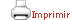Teaching plan for the course unit

 CloseGeneral information

Course unit name: Applied Harmonic Analysis

Course unit code: 568183

Coordinator: Francesc Xavier Massaneda Clares

Department: Department of Mathematics and Computer Science

Credits: 6

Single program: S

 Estimated learning time Total number of hours 150

 Face-to-face and/or online activities 60
 -  Lecture Face-to-face 30 (In-class or online) -  Lecture with practical component Face-to-face 15 (In-class or online) -  Laboratory session Face-to-face 15 (In-class or online)
 Supervised project 20
 Independent learning 70

 Recommendations

 Students should have completed introductory courses in Calculus, Functional Analysis and Measure Theory. Further recommendations Students should have some knowledge of computer programming.

 Competences to be gained during study

 — Capacity to understand the concepts and rigorous proofs of fundamental theorems of certain specific areas of mathematics.— Capacity to apply the results and acquired techniques to solve complex problems in certain areas of mathematics in academic or professional contexts.— Ability to prepare and develop logical mathematical reasoning and to identify errors in incorrect reasoning.— Capacity to construct, interpret, analyze and validate mathematical models developed to simulate real situations.— Ability to enunciate and verify statements, and to communicate the acquired mathematical knowledge orally or in writing.— Capacity to select and use software tools to address problems related to mathematics.

 Learning objectives
 Referring to knowledge — To know and understand some of the classical results on Fourier series and on the Fourier transform.— To provide an introduction to other parts of harmonic analysis which are relevant in applications, such as the short-time Fourier transform, Gabor frames, the theory of wavelets and the Radon transform.— To acquire a broad knowledge of the applications of Fourier theory in image processing and digital signal analysis, learning to compress an image or filter a noisy signal.— To acquire basic research skills in the field of harmonic analysis.

 Teaching blocks

1. Fourier Series; Orthonormal basis and L2 theory; Convergence of the series

2. Fourier transform; Shannon Sampling theorem

3. Short-time Fourier transform; Gabor frames

4. Convolution operators; Fourier multipliers

5. Radon transform and Computed Tomography

6. Wavelets.

7. MATLAB

7.1. Introduction to MATLAB

7.2. Basic digital signal processing

7.3. The Z transform and filter design

7.4. The discrete Fourier transform and the Fast Fourier Transform

7.5. The Radon transform: the backprojection algorithm

7.6. The Radon transform: Fourier methods

7.7. Compressed sensing

7.8. Wavelets

 Teaching methods and general organization

 Different topics will be presented in the theory lectures. For each topic, a collection of problems will be handed out. Students will prepare these exercises at home and present the solutions either in class or in a written document. In the second part of the course they will also be assigned a series of programming exercises in MATLAB/OCTAVE, to apply digital processing techniques to images and sounds. In case of mixed teaching (half in-class and half online) the theoretical part of the course will be posted online. The in-class sessions will be devoted to review the most important aspects of the theory, to solve problems and exercises and to the computer labs. The methodology of the subject will include actions to enhance self-efficacy, reduce insecurity and combat gender stereotypes. Likewise, we will try to respect and promote diversity within students, both in acquired skills and in interests and sensibilities.

 Official assessment of learning outcomes

 The continuous evaluation of the course has two parts:Activity 1 (A1): Resolution of exercises given in the first part of the courseActivity 2 (A2): MATLAB/OCTAVE assignments given in the second part of the course If both A1 and A2 are 6 or more (out of 10), the final grade will be the average (A1+A2)/2. If A1 or A2 is less than 6, there will be a final exam F. The final grade will be the maximum between (A1+A2)/2 and F.     Examination-based assessment The single evaluation consists of a final examination with theoretical and practical questions (exercises and MATLAB/OCTAVE programs). Re-evaluation:In order to be eligible for re-evaluation, students must have obtained a final grade of at least 3.5/10.